#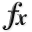N-D Test Functions G¶

class go_benchmark.Gear(dimensions=4)

Gear test objective function.

This class defines the Gear global optimization problem. This is a multimodal minimization problem defined as follows: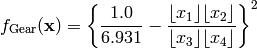Here,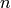represents the number of dimensions and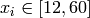for.

Global optimum:for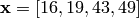, where the various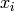may be permuted.

class go_benchmark.Giunta(dimensions=2)

Giunta test objective function.

This class defines the Giunta global optimization problem. This is a multimodal minimization problem defined as follows:Here,represents the number of dimensions and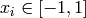for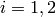.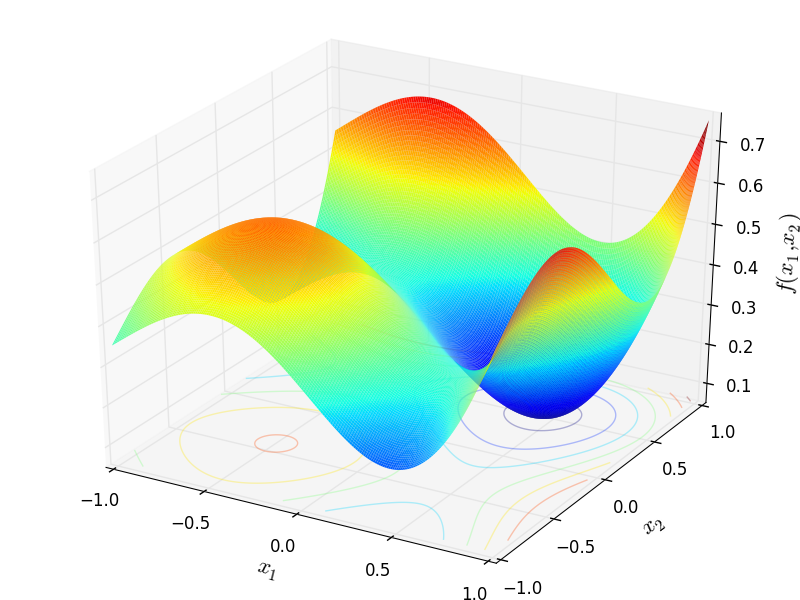Two-dimensional Giunta function

Global optimum: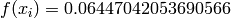for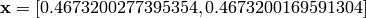class go_benchmark.GoldsteinPrice(dimensions=2)

Goldstein-Price test objective function.

This class defines the Goldstein-Price global optimization problem. This is a multimodal minimization problem defined as follows: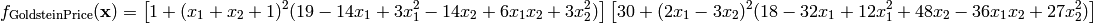Here,represents the number of dimensions and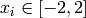for.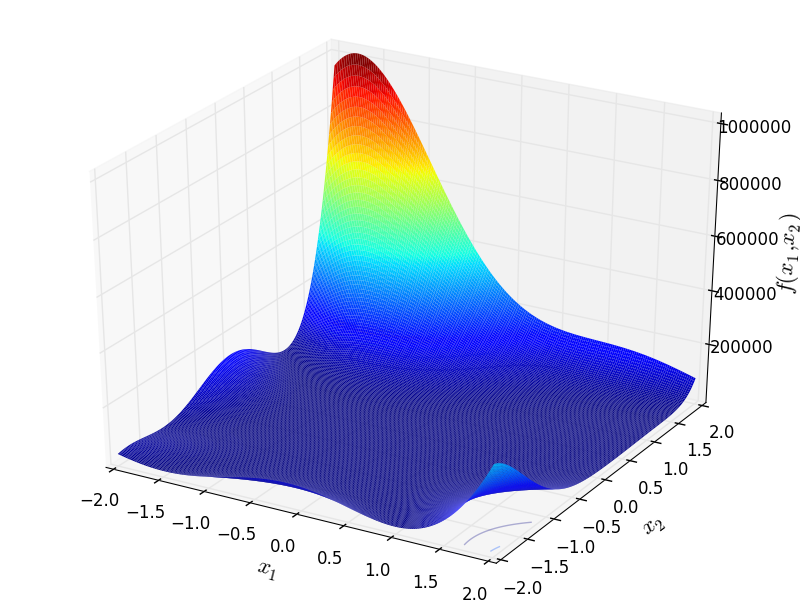Two-dimensional Goldstein-Price function

Global optimum: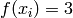forclass go_benchmark.Griewank(dimensions=2)

Griewank test objective function.

This class defines the Griewank global optimization problem. This is a multimodal minimization problem defined as follows:Here,represents the number of dimensions and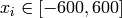for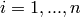.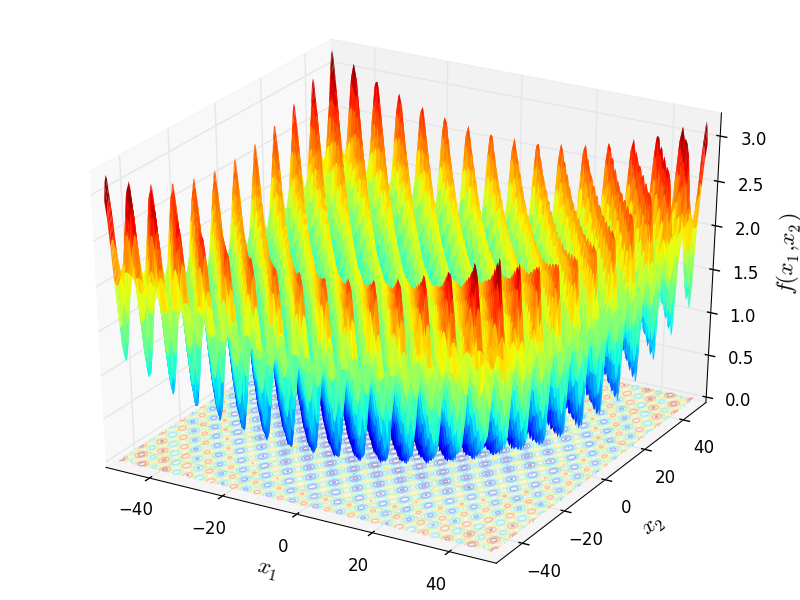Two-dimensional Griewank function

Global optimum: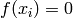for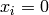forclass go_benchmark.Gulf(dimensions=3)

Gulf test objective function.

This class defines the Gulf global optimization problem. This is a multimodal minimization problem defined as follows: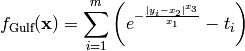Where, in this exercise:Here,represents the number of dimensions andfor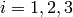.

Global optimum:for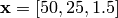#### Previous topic

N-D Test Functions F

#### Next topic

N-D Test Functions H